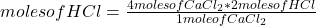If you have 4 moles of CaCl2 how many moles of HCI can you make Equation- CaCl2 +2H2O= Ca(OH)2+2HCl

Question

If you have 4 moles of CaCl2 how many moles of HCI can you make

Equation- CaCl2 +2H2O= Ca(OH)2+2HCl

in progress 0
5 months 2021-08-16T04:33:23+00:00 1 Answers 3 views 0

If you hace 4 moles of CaCl₂, you can make 8 moles of HCl.

Explanation:

The balanced equation is

CaCl₂ + 2 H₂O → Ca(OH)₂ + 2 HCl

By reaction stoichiometry (that is, the relationship between the amount of reagents and products in a chemical reaction) , the following amounts of each compound participate in the reaction:

• CaCl₂: 1 mole
• H₂O: 2 moles
• Ca(OH)₂: 1 mole
• HCl: 2 moles

Then you can apply the following rule of three: if by stoichiometry 1 mole of CaCl₂ forms 2 moles of HCl, 4 moles of CaCl₂ form how many moles of HCl?moles of HCl= 8

If you hace 4 moles of CaCl₂, you can make 8 moles of HCl.